Skip to main content

# 3.10: Additional Problems

Exercise $$\PageIndex{1}$$

For each of the following hand-drawn cartoons of IR spectra, identify the bond(s) that correspond to the indicated peaks.

i.ii.iii.iv.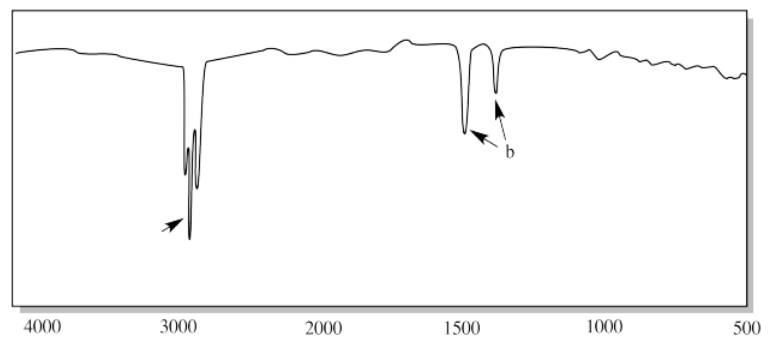v.vi.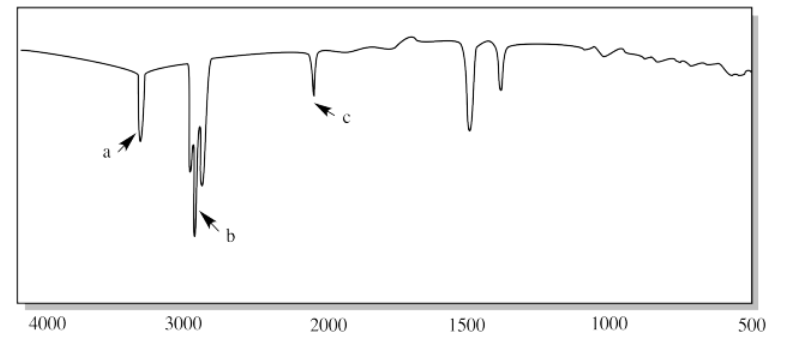vii.viii.ix.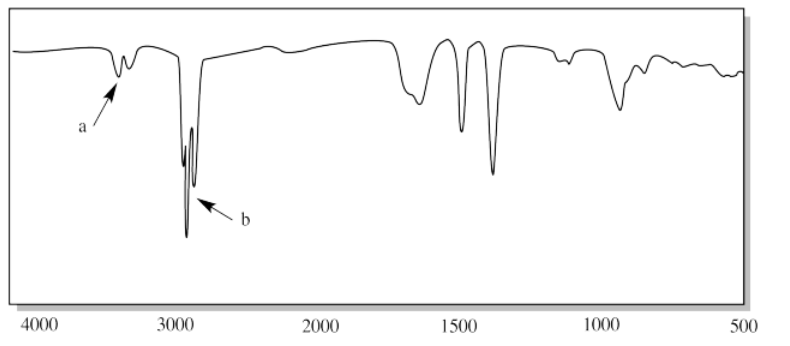x.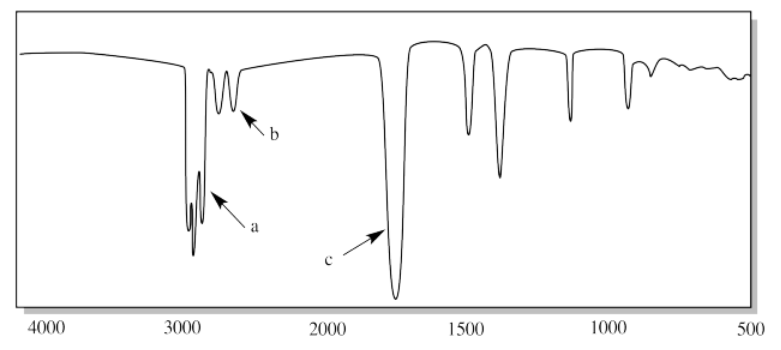xi.xii.xiii.Answer

i. a) O-H b) sp3 C-H c) C-O

ii. a) sp2 C-H b) sp3 C-H c) C=C-H (oop bend)

iii. a) N-H (two of them) b) C=O c) C-N

iv. a) sp3 C-H b) H-C-H (CH2 bend)

v. a) sp3 C-H b) C=O c) H-C-H (CH2 bend)

vi. a) sp C-H b) sp3 C-H c) C=C

vii. a) N-H b) sp3 C-H

viii. a) C=O b) C-O

ix. a) N-H (two of them) b) sp3 C-H

x. a) sp3 C-H b) aldehyde C-H c) C=O

xi. a) H-C-H (CH2 bend) b) C-O

xii. a) O-H (very broad in CO2H) b) sp3 C-H c) C=O d) C-O

xiii. a) sp2 C-H b) sp3 C-H c) aromatic overtones d) C=C

Exercise $$\PageIndex{2}$$

For each of the following hand-drawn cartoons of IR spectra, identify the functional group suggested by the spectrum.

a)b)c)d)e)f)g)h)i)j)k)Answer

a) The rounded OH peak near 3300 cm-1 and the strong C-O peak near 1100 cm-1 suggest an alcohol.

b) The sharp CH peaks below 3000 cm-1, the weak CH2 bending modes near 1500 cm-1 and the absence of any other features suggest an alkane.

c) The strong C=O peak near 1700 cm-1 and the strong C-O peak near 1100 cm-1 suggest an ester.

d) The strong C=O peak near 1700 cm-1 and the absence of additional features other than those associated with saturated hydrocarbons suggest a ketone.

e) The CH peak above 3000 cm-1 and the strong oop bending modes below 1000 cm-1 suggest an alkene. The presence of two oop bending peaks may point to a terminal alkene (C=CH2).

f) The sharp N-H peaks near 3200 cm-1 and the strong C=O peak near 1600 cm-1 suggest an amide. the presence of two N-H peaks rather than one points to a primary amide (O=C-NH2).

g) The small, triangular "sharktooth" peak near 3200 cm-1 suggests an amine. With just one N-H peak, this is probably a primary amine (R-NH2).

h) The strong C-O peak near 1100 cm-1 suggests an ether.

i) The C-H peaks above 3000 cm-1 and the oop bending modes below 1000 cm-1 certainly suggest double bonds. The progression of tooth-like "aromatic overtones" between 1600 and 2000 cm-1 strongly indicates a substituted benzene.

j) The small, triangular "sharktooth" peaks near 3200 cm-1 suggest an amine. With two N-H peaks, this is probably a secondary amine (R2NH).

l) The broad, deep OH peak between 3300 cm-1 and 2600 cm-1 and the C=O peak near 1700 cm-1 suggests a carboxylic acid. The O-H peak of a carboxylic acid is often missed; it is moved to lower frequency by hydrogen bonding.

Exercise $$\PageIndex{3}$$

a) Identify at least three important peaks/bonds in each of the following IR spectra.

b) Identify the functional group present in each of these samples. See the Functional Group Section

for help.

c) Draw a possible structure for each of these compounds (there may be many, many correct answers).

problem i)problem ii)problem iii)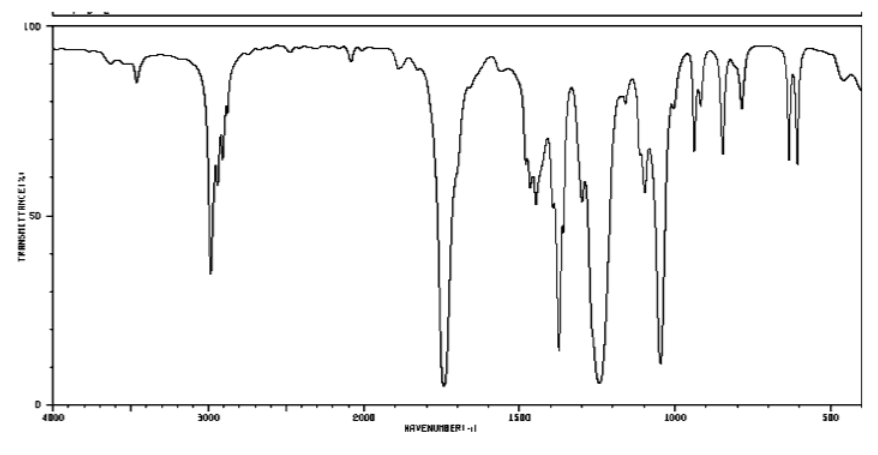problem iv)problem v)Answer

i)

a)

3200 cm-1 (sharp): N-H

2900 cm-1 (sharp): Csp3-H

1600 cm-1 (broad): probably NH2 bending

b) A primary amine.

c)

ii)

a)

3400 cm-1 (strong, broad): O-H

2900 cm-1 (sharp): Csp3-H

1050 cm-1 (strong): probably C-O

b) Alcohol.

c)

iii)

a)

2900 cm-1 (sharp): Csp3-H

1700 cm-1 (strong): C=O

1200 and 1000 cm-1 (strong & medium): C-O

b) Ester

c)

iv)

a)

3050 cm-1 (sharp): Csp2-H

1700 cm-1 (strong): C=O

1200 and 1000 cm-1 (strong & medium): C-O

b) Ester; probably contains an aromatic as well

c)

v)

a)

2750 and 2650 cm-1 (medium, sharp): C-H of an aldehyde

1700 cm-1 (strong): C=O

Below 1000 cm-1 (strong & medium): oop bends

b) Aldehyde; probably contains an aromatic as well

Exercise $$\PageIndex{4}$$

Sketch an approximate IR spectrum for each of the following compounds:Exercise $$\PageIndex{5}$$

The oop bends are sometimes useful in distinguishing substitution pattrens around a benzene ring. Using the spectra of o-, m-, and p-xylene, formulate some guidelines about what the oop bends look like when substituents are one, two or three carbons away on a benzene ring.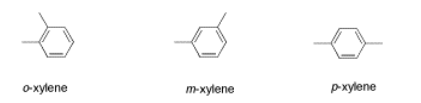Figure $$\PageIndex{1}$$: IR spectrum of m-xylene.

Source: SDBSWeb : http://riodb01.ibase.aist.go.jp/sdbs/ (National Institute of Advanced Industrial Science and Technology of Japan, 14 July 2008)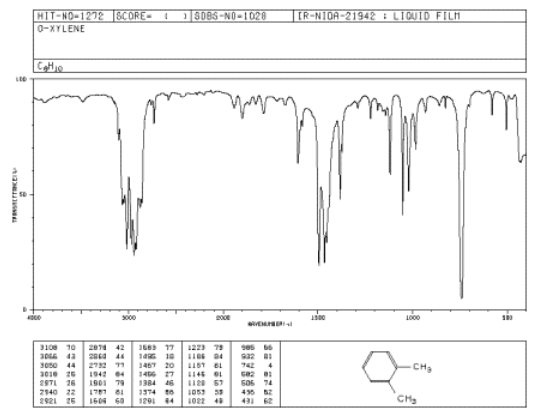Figure $$\PageIndex{2}$$: IR spectrum of o-xylene.

Source: SDBSWeb : http://riodb01.ibase.aist.go.jp/sdbs/ (National Institute of Advanced Industrial Science and Technology of Japan, 14 July 2008)Figure $$\PageIndex{3}$$: IR spectrum of p-xylene.

Source: SDBSWeb : http://riodb01.ibase.aist.go.jp/sdbs/ (National Institute of Advanced Industrial Science and Technology of Japan, 14 July 2008